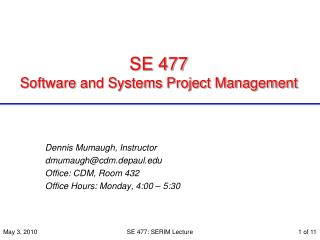DownloadDownload PresentationSE 477 Software and Systems Project Management

# SE 477 Software and Systems Project Management

Download Presentation## SE 477 Software and Systems Project Management

- - - - - - - - - - - - - - - - - - - - - - - - - - - E N D - - - - - - - - - - - - - - - - - - - - - - - - - - -
##### Presentation Transcript

1. SE 477 Software and Systems Project Management Dennis Mumaugh, Instructor dmumaugh@cdm.depaul.edu Office: CDM, Room 432 Office Hours: Monday, 4:00 – 5:30 SE 477: SERIM Lecture

2. Software Engineering Risk Model (SERIM) SE 477: SERIM Lecture

3. Software Engineering Risk Model (SERIM) SE 477: SERIM Lecture Applicable for small and medium sized organizations. Primarily qualitative with quantitative analysis. Applicable to full life-cycle including maintenance phase. Maintainable during project development. Compatible with any development model, such as spiral, waterfall, joint application design, recent application design, incremental, or prototyping. SERIM uses risk questions to derive numerical probabilities for a set of risk factors and analyzes them using a simple spreadsheet.

4. SERIM Terminology • Risk Elements – Technical, Cost, Scheduling problems. • Risk Factors – more specific subcategories of the three general risk elements (for example organizational risk). A risk factor can be related to one or more risk elements. SERIM identifies ten primary risk factors of: organization, estimation, monitoring, development, tools, risk culture, usability, correctness, reliability, and personnel. Each software risk factor can have an influence on each risk element. That influence can be categorized as low, medium, or high. SE 477: SERIM Lecture

5. SERIM Terminology The impact of each risk factor on each risk element. The effect of risk factors on each risk categories. SE 477: SERIM Lecture

6. Risk questions • One approach to assessing risk is to use risk questions to measure risk factors. • Answers to the questions can be recorded in a yes-or-no format (0 or 1) or as a numerical range of possible responses. • Response ranges can use any numerical value from 0 through 1. For example, a response range could be defined as none = 0, little = 0.2, some = 0.5, most = 0.8, and all = 1.0. The relationship of risk questions to risk factors and risk elements. SE 477: SERIM Lecture

7. Organizational questions O1. Are you using or do you plan to use experienced software managers? O2. Has your company been producing software similar to this in the past? O3. Is there a documented organizational structure either in place or planned? O4. Is the organizational structure stable? O5. What is the confidence level of your management team? O6. Does good communication exist between different organizations supporting the development of the software project? O7. Are software configuration management functions being performed? O8. Are software quality functions being performed? Scores Devise a numerical range of responses: For example in question O1: 1= Mgrs with little or no SE experience. 0.5 = Mix of experienced and non-experienced managers. 0 = Only experienced managers. SE 477: SERIM Lecture

8. Calculating P(An) Example Organizational success: 2. Calculate Imp / Impact Total. 3. Calculate % of Impact X success. 1. Sum the impacts. SE 477: SERIM Lecture

9. Quantitative Risk Analysis • The probability of a successful project P(A) is derived using the following equation where E = the number of risk elements. This equation assumes that all risk elements are equal in weight. P(A) is the probability of a successful project. • If different risk elements have different impacts (weights), then:P(A) =w1P(A1) + w2P(A2) + w3P(A3), where w1 is a positive number and w1 +w2 + w3 = 1. SE 477: SERIM Lecture

10. Example: • 5 risk elements at the following probabilities for success:A1 = 0.9, A2 = 0.75, A3 = 0.85, A4 = 0.6, A5 = 0.95 • Risk elements weighted as: w1 = 0.2, w2 = 0.4, w3 = 0.1, w4 = 0.1, w5 = 0.2 • Probability for successP(A) = (0.9*0.2) + (0.75*0.4) + (0.85*0.1) + (0.6*0.1) + (0.95*0.2) P(A) = 0.815 • Question: Should we proceed?????? SE 477: SERIM Lecture

11. Journal Exercises • Discuss SERIM. [No, it is not an Italian vending machine company with a good cup of coffee.] What is it good for? How does it work? SE 477: SERIM Lecture